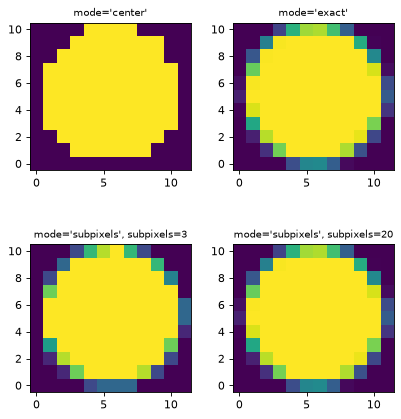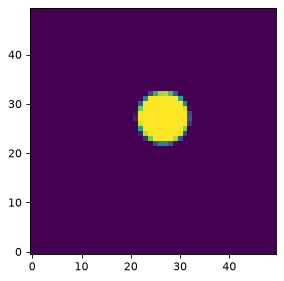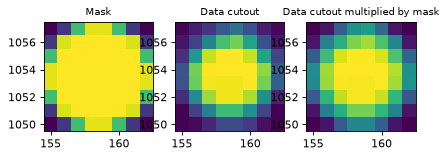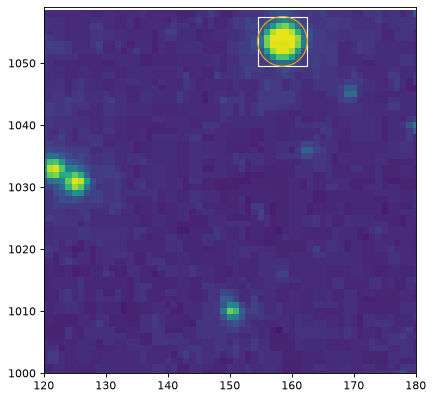## Defining a region mask within its bounding box¶

For aperture photometry, a common operation is to compute, for a given image and region, a mask or array of pixel indices defining which pixels (in the whole image or a minimal rectangular bounding box) are inside and outside the region.

All PixelRegion objects have a to_mask() method that returns a RegionMask object that contains information about whether pixels are inside the region, and can be used to mask data arrays:

>>> from regions.core import PixCoord
>>> from regions.shapes.circle import CirclePixelRegion
>>> center = PixCoord(4., 5.)
>>> reg = CirclePixelRegion(center, 2.3411)
array([[0., 1., 1., 1., 0.],
[1., 1., 1., 1., 1.],
[1., 1., 1., 1., 1.],
[1., 1., 1., 1., 1.],
[0., 1., 1., 1., 0.]])


The mask data contains floating point that are between 0 (no overlap) and 1 (overlap). By default, this is determined by looking only at the central position in each pixel, and:

>>> reg.to_mask()


is equivalent to:

>>> reg.to_mask(mode='center')


but other modes are available:

• mode='exact': the overlap is determined using the exact geometrical overlap between pixels and the region. This is slower than using the central position, but allows partial overlap to be treated correctly.

• mode='subpixels': the overlap is determined by sub-sampling the pixel using a grid of sub-pixels. The number of sub-pixels to use in this mode should be given using the subpixels argument.

Here are what the different modes look like:

import matplotlib.pyplot as plt
from regions.core import PixCoord
from regions.shapes.circle import CirclePixelRegion

center = PixCoord(26.6, 27.2)
reg = CirclePixelRegion(center, 5.2)

plt.figure(figsize=(6, 6))

plt.subplot(2, 2, 1)
plt.title("mode='center'", size=9)
interpolation='nearest', origin='lower')

plt.subplot(2, 2, 2)
plt.title("mode='exact'", size=9)
interpolation='nearest', origin='lower')

plt.subplot(2, 2, 3)
plt.title("mode='subpixels', subpixels=3", size=9)
interpolation='nearest', origin='lower')

plt.subplot(2, 2, 4)
plt.title("mode='subpixels', subpixels=20", size=9)
interpolation='nearest', origin='lower')As we’ve seen above, the RegionMask objects have a data attribute that contains a Numpy array with the mask values. However, if you have for example a circular region with a radius of 3 pixels at a pixel position of (1000, 1000), it would be inefficient to store a mask that has a size larger than this, so instead we store the mask using the minimal array that contains the mask, and the RegionMask objects also include a bbox attribute that is a BoundingBox object used to indicate where the mask should be applied in an image.

## Defining a region mask within an image¶

RegionMask objects also have a number of methods to make it easy to use the masks with data. The to_image() method can be used to obtain an image of the mask in a 2D array of the given shape. This places the mask in the correct place in the image and deals properly with boundary effects. For this example, let’s place the mask in an image with shape (50, 50):

import matplotlib.pyplot as plt
from regions.core import PixCoord
from regions.shapes.circle import CirclePixelRegion

center = PixCoord(26.6, 27.2)
reg = CirclePixelRegion(center, 5.2)

plt.figure(figsize=(4, 4))
shape = (50, 50)
interpolation='nearest', origin='lower')## Making image cutouts and multiplying the region mask¶

The cutout() method can be used to create a cutout from the input data over the mask bounding box, and the multiply() method can be used to multiply the aperture mask with the input data to create a mask-weighted data cutout. All of these methods properly handle the cases of partial or no overlap of the aperture mask with the data.

These masks can be used as the building blocks for photometry, which we demonstrate with a simple example. We start off by getting an example image:

>>> from astropy.io import fits
>>> from astropy.utils.data import get_pkg_data_filename
>>> filename = get_pkg_data_filename('photometry/M6707HH.fits')
>>> pf = fits.open(filename)
>>> hdu = pf


We then define the aperture:

>>> from regions.core import PixCoord
>>> from regions.shapes.circle import CirclePixelRegion
>>> center = PixCoord(158.5, 1053.5)
>>> aperture = CirclePixelRegion(center, 4.)


We convert the aperture to a mask and extract a cutout from the data, as well as a cutout with the data multiplied by the mask:

>>> mask = aperture.to_mask(mode='exact')
>>> pf.close()


We can take a look at the results to make sure the source overlaps with the aperture:

.. doctest-skip::

>>> import matplotlib.pyplot as plt
>>> plt.subplot(1, 3, 1)
...            interpolation='nearest', origin='lower',
>>> plt.subplot(1,3,2)
>>> plt.title("Data cutout", size=9)
>>> plt.imshow(data, cmap=plt.cm.viridis,
...            interpolation='nearest', origin='lower',
>>> plt.subplot(1,3,3)
>>> plt.title("Data cutout multiplied by mask", size=9)
>>> plt.imshow(weighted_data, cmap=plt.cm.viridis,
...            interpolation='nearest', origin='lower',We can also use the RegionMask bbox attribute to look at the extent of the mask in the image:

ax = plt.subplot(1, 1, 1)
ax.imshow(hdu.data, cmap=plt.cm.viridis,
interpolation='nearest', origin='lower')>>> import numpy as np